Current through wire XY of circuit shown is :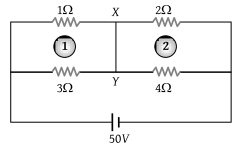(1) 1 A

(2) 4 A

(3) 2 A

(4) 3 A

Concept Questions :-

Combination of resistors
High Yielding Test Series + Question Bank - NEET 2020

Difficulty Level:

12 cells each having same emf are connected in series with some cells wrongly connected. The arrangement is connected in series with an ammeter and two cells which are in series. Current is 3 A when cells and battery aid each other and is 2 A when cells and battery oppose each other. The number of cells wrongly connected is

(1) 4

(2) 1

(3) 3

(4) 2

Concept Questions :-

Grouping of cells
High Yielding Test Series + Question Bank - NEET 2020

Difficulty Level:

Following figure shows cross-sections through three long conductors of the same length and material, with square cross-section of edge lengths as shown. Conductor B will fit snugly within conductor A, and conductor C will fit snugly within conductor B. Relationship between their end to end resistance is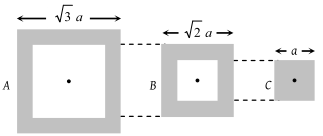(1) RA = RB = RC

(2) RA > RB > RC

(3) RA < RB < R

(4) Information is not sufficient

Concept Questions :-

Derivation of Ohm's law
High Yielding Test Series + Question Bank - NEET 2020

Difficulty Level:

In the following star circuit diagram (figure), the equivalent resistance between the points A and H will be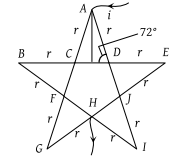(1) 1.944 r

(2) 0.973 r

(3) 0.486 r

(4) 0.243 r

Concept Questions :-

Combination of resistors
High Yielding Test Series + Question Bank - NEET 2020

Difficulty Level:

In the adjoining circuit diagram each resistance is of 10 Ω. The current in the arm AD will be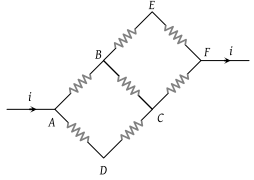1. $\frac{2i}{5}$

2. $\frac{3i}{5}$

3. $\frac{4i}{5}$

4. $\frac{i}{5}$

Concept Questions :-

Combination of resistors
High Yielding Test Series + Question Bank - NEET 2020

Difficulty Level:

In the circuit of adjoining figure the current through 12 Ω resister will be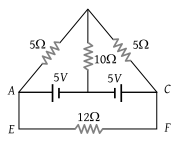(1) 1 A

(2) $\frac{1}{5}A$

(3) $\frac{2}{5}A$

(4) 0 A

Concept Questions :-

Combination of resistors
High Yielding Test Series + Question Bank - NEET 2020

Difficulty Level:

The reading of the ideal voltmeter in the adjoining diagram will be :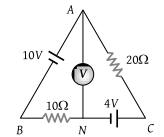1. 4 V

2. 8 V

3. 12 V

4. 14 V

Concept Questions :-

Combination of resistors
High Yielding Test Series + Question Bank - NEET 2020

Difficulty Level:

The resistance of the series combination of two resistance is S. When they are joined in parallel the total resistance is P. If S = nP, then the minimum possible value of n is :

(1) 4

(2) 3

(3) 2

(4) 1

Concept Questions :-

Combination of resistors
High Yielding Test Series + Question Bank - NEET 2020

Difficulty Level:

Which of the adjoining graphs represents ohmic resistance :

(1)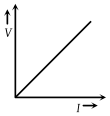(2)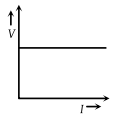(3)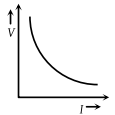(4)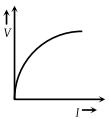Concept Questions :-

Derivation of Ohm's law
High Yielding Test Series + Question Bank - NEET 2020

Difficulty Level:

Variation of current passing through a conductor as the voltage applied across its ends as varied is shown in the adjoining diagram. If the resistance (R) is determined at points A, B, C and D, we will find that :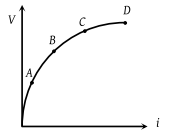(1) RC = RD

(2) RB > RA

(3) RC > RB

(4) None of these

Concept Questions :-

Derivation of Ohm's law